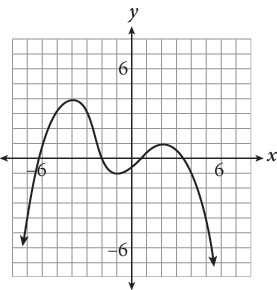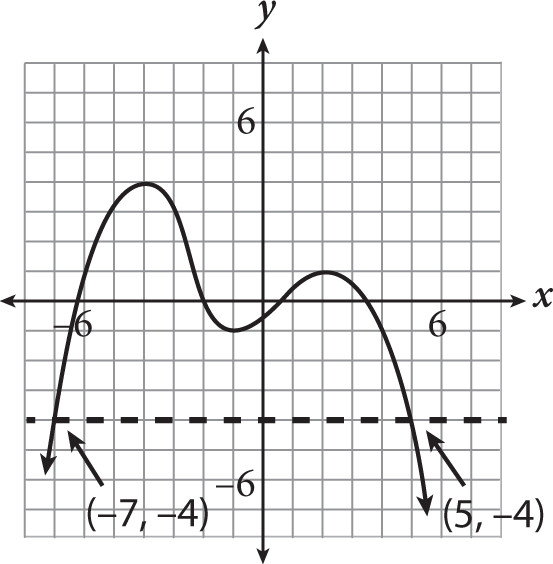# SAT Math Multiple Choice Question 514: Answer and Explanation

### Test Information

Question: 514

4.The graph of a polynomial function p(x) is shown above. For what values of x does p(x) = -4?

• A. –1
• B. 4
• C. –7 and 5
• D. –7, 4, and 5

Explanation:

C

Difficulty: Easy

Category: Passport to Advanced Math / Functions

Strategic Advice: Understanding the language of functions will make answering this question very simple. Another way of saying For what values of x does f(x) = –4? is What is the x-value when y = –4?

Getting to the Answer: Draw a line across the graph at y = –4 and find the x-coordinates of any points that hit your line.The line hits the graph at x = –7 and at x = 5.